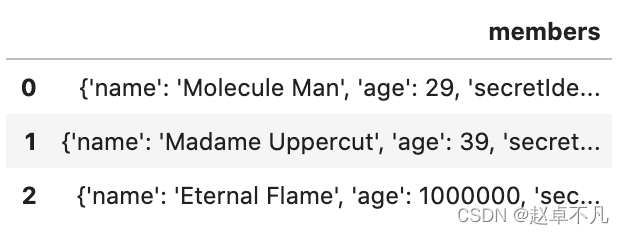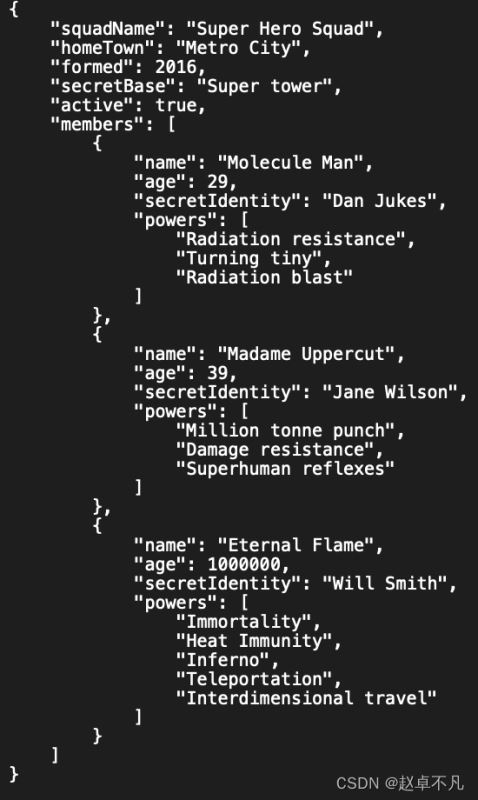﻿ Python中优雅处理JSON文件的方法实例_python_脚本之家
python# Python中优雅处理JSON文件的方法实例

JSON是一种轻量级的数据交换格式,JSON采用完全独立于语言的文本格式，但是也使用了类似于C语言家族的习惯,这篇文章主要给大家介绍了关于Python中优雅处理JSON文件的相关资料,需要的朋友可以参考下

## 2. 什么是JSON文件?

JSON（Java Script Object Notation）是一种流行的文件格式，主要用于在web应用程序中存储和传输数据。如果我们经常和数据打交道，那么一定或多或少遇到过JSON格式的文件，因此我们有必要来学习如何读取和写入JSON。JSON结构看起来和Python中的字典非常类似。需要注意的是，JSON格式通常是由key: 结对组成,其中key是字符串形式,value是字符串、数字、布尔值、数组、对象或null。## 3. 使用Python处理JSON文件

### 3.1. 将JSON文件读取为字典类型

```import json

with open('superheroes.json') as f:

# Output: dict_keys(['squadName', 'homeTown', 'formed', 'secretBase', 'active', 'members'])
```

### 3.2. 将JSON文件读取为Pandas类型

```import pandas as pd
``````df1 = pd.read_json('https://mdn.github.io/learning-area/javascript/oojs/json/superheroes.json')
```

### 3.3. 使用Pandas读取嵌套JSON类型```df['members'].apply(pd.Series)
``````df = pd.concat([df['members'].apply(pd.Series), df.drop('members', axis = 1)], axis = 1)
```

```def test2():
with open('superheroes.json') as f:
print(out)
```

• record_path为我们希望拆分的列的名字
• meta为列名的list，为我们输出的次序```pd.json_normalize(superHeroSquad,
record_path = ['members'],
meta = ['squadName', 'homeTown', 'formed', 'secretBase', 'active'],
meta_prefix = 'members_')
```### 3.4. 访问特定位置的数据```superHeroSquad['members']['secretIdentity']
```### 3.5. 导出JSON

```#update secret identity of Eternal Flame
with open('superheroes.json', 'w') as file:
```

```df.to_json('superheroes.json')
```

### 3.6. 格式化输出```with open('superheroes.json', 'w') as file:
```### 3.7. 输出字段排序

```with open('superheroes.json', 'w') as file:
json.dump(superHeroSquad, file, indent = 4, sort_keys = True)
```## 4.总结

• JSON文件通常由key:结对组成，这里key通常为字符串格式，value一般为字符串，数字，布尔，数组，对象或者null
• Python有内置函数可以方便的读取JSON文件转化为Python中的字典类型或者Pandas可以处理的类型